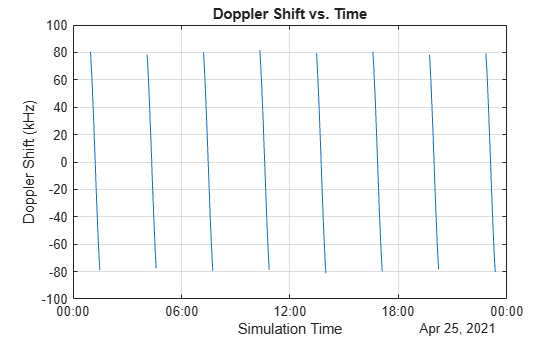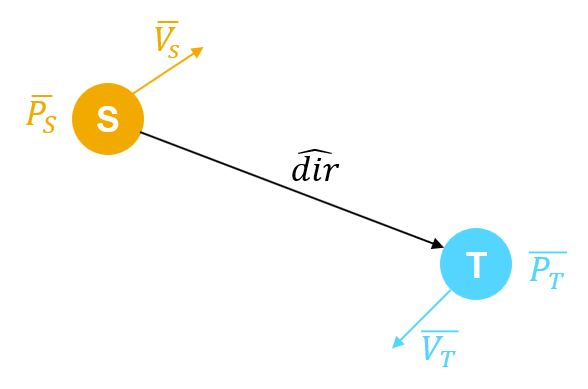# dopplershift

Calculate Doppler shift at target asset in satellite scenario

Since R2023a

## Syntax

``shift = dopplershift(source,target)``
``[shift,timeOut] = dopplershift(source,target)``
``[shift,timeOut,info] = dopplershift(source,target)``
``[___] = dopplershift(___,timeIn)``
``[___] = dopplershift(___,Frequency=freq)``

## Description

example

````shift = dopplershift(source,target)` calculates the history of Doppler shifts at the `target` asset corresponding to the `source` asset. Both `source` and `target` must belong to the same `satelliteScenario` object. If the value of `AutoSimulate` property of the `satelliteScenario` object is `true`, the `dopplershift` function returns the Doppler shift history from the value of `StartTime` to the value of `StopTime`. Otherwise, it returns the Doppler shift history from the value of `StartTime` to the value of `SimulationTime`.```
````[shift,timeOut] = dopplershift(source,target)` returns the sample time history `timeOut`.```
````[shift,timeOut,info] = dopplershift(source,target)` returns a structure, `info`, containing the Doppler rate and relative velocity information. ```
````[___] = dopplershift(___,timeIn)` calculates Doppler shift at the specified datetime `timeIn`. When using this syntax, `dopplershift` sets the second dimension of `shift`, `timeOut`, and the fields of `info` to `1`. In this case, the ``` DopplerRate``` field of `info` is empty.```
````[___] = dopplershift(___,Frequency=freq)` additionally specifies one or more carrier frequencies at which to calculate Doppler shift. NoteThe `dopplershift` function uses the Geocentric Celestial Reference Frame (GCRF) coordinate system to perform all calculations. ```

## Examples

collapse all

Determine the Doppler shift, the Doppler rate, the relative velocity, and the corresponding time history at a ground station. The source is a satellite that transmits on a carrier frequency of `14` GHz.

Specify the start time and the stop time of the satellite scenario.

```startTime = datetime(2021,4,25); % April 25, 2021, 12:00 AM UTC stopTime = datetime(2021,4,26); % April 26, 2021, 12:00 AM UTC```

Set the sample time in seconds.

`sampleTime = 60; `

Create a `satelliteScenario` object.

`sc = satelliteScenario(startTime,stopTime,sampleTime);`

Add a satellite to the scenario.

```semiMajorAxis = 10000000; % In meters eccentricity = 0; inclination = 0; % In degrees rightAscensionOfAscendingNode = 0; % In degrees argumentOfPeriapsis = 0; % In degrees trueAnomaly = 0; % In degrees sat = satellite(sc,semiMajorAxis,eccentricity,inclination, ... rightAscensionOfAscendingNode,argumentOfPeriapsis,trueAnomaly);```

Add a default ground station to the scenario.

`gs = groundStation(sc);`

Determine the Doppler shift, the Doppler rate, and the relative velocity, as well as the corresponding time history.

```carrierFrequency=14e9; [frequencyShift,timeOut,dopplerInfo] = dopplershift(sat,gs,Frequency=carrierFrequency); frequencyRate = dopplerInfo.DopplerRate; relativeVelocity = dopplerInfo.RelativeVelocity;```

Plot the Doppler shift at the ground station.

```plot(timeOut,frequencyShift(1,:)/1e3) % Doppler shift in kilohertz (kHz) xlim([timeOut(1) timeOut(end)]) title("Doppler Shift vs. Time") xlabel("Simulation Time") ylabel("Doppler Shift (kHz)") grid on```## Input Arguments

collapse all

Source asset from scenario, specified as a `Satellite` object, `GroundStation` object, `Transmitter` object, array of `Satellite` objects, array of `GroundStation` objects, or array of `Transmitter` objects.

Target asset from scenario, specified as a `Satellite` object, `GroundStation` object, `Receiver` object, array of `Satellite` objects, array of `GroundStation` objects, or array of `Receiver` objects.

Time at which the function calculates the Doppler shift, specified as a datetime scalar. If `timeIn` does not specify a time zone, the function uses Coordinated Universal Time (UTC).

Data Types: `datetime`

Carrier frequency at which to calculate Doppler shift, specified as a scalar or vector of nonnegative real numbers, in hertz or `-1`. Specify `-1` to use the default carrier frequency value for each source asset.

If the `freq` value is `-1` and `source` is a `Satellite` or `GroundStation`, the carrier frequency is 14 gigahertz (GHz) . If the `freq` value is `-1` and `source` is a `Transmitter`, the carrier frequency is the `Frequency` property value of the `Transmitter` object.

Data Types: `double`

## Output Arguments

collapse all

History of Doppler shift, in hertz, returned as a scalar, vector, matrix, or 3-D array. When the `source` and `target` cannot access each other, the corresponding `shift` value is `NaN`. These tables show how this output changes based on the different input argument sizes and combinations.

• `timeIn` not specified

 `source` `target` `shift` Description scalar scalar row vector Doppler shift history, in which each element represents the Doppler shift between `source` and `target` at a specific time sample. scalar vector matrix Each row represents the Doppler shift history between source and an asset in` target`. The row corresponds to the position of the asset in `target`. vector scalar matrix Each row represents the Doppler shift history between `target` and an asset in `source`. The row corresponds to the position of the asset in `source`. vector vector matrix Each row represents the Doppler shift history between a pair of `source` and `target` assets. The row corresponds to the position of the assets in `source` and `target`.

• `timeIn` specified

 `source` `target` `shift` Description scalar scalar scalar Doppler shift at the specified datetime `timeIn`. scalar vector column vector Each element represents the Doppler shift between ```source ``` and the corresponding asset in `target` at the specified datetime `timeIn`. vector scalar column vector Each element represents the Doppler shift from the corresponding asset in `source` to `target` at the specified datetime `timeIn`. vector vector column vector Each element represents the Doppler shift between the corresponding pair of `source` and `target` assets at the specified datetime `timeIn`.

If you specify freq as a vector, shift has a third dimension in which each page is the output of the corresponding combination of `source`, `target`, and `timeIn` from the previous tables for the corresponding carrier frequency in `freq`.

Data Types: `double`

Time samples between the start and stop time of the scenario, returned as a scalar or vector.

If `timeIn` does not specify a time zone and the `AutoSimulate` property the `satelliteScenario` object is `true`, the `dopplershift` function returns the time sample history from `StartTime` to `StopTime`. Otherwise, it returns the time sample history from the value of `StartTime` to the value of `SimulationTime`.

Data Types: `datetime`

History of Doppler rate and relative velocity, returned as a structure that contains these fields.

• `DopplerRate` — The Doppler rate history, returned as a vector, matrix, or 3-D array. The format of `DopplerRate` is similar to `shift` except that the number of columns equals the number of time samples minus 1. When `source` and `target` cannot see each other, the corresponding `DopplerRate` value is `NaN`.

• `RelativeVelocity` — The history of relative velocity of `source` and `target` in the direction from `source` to `target`, returned as a scalar, vector, or matrix. The number of rows in the matrix is the greater number between the `source` and ` target` assets. When `source` and `target` cannot access each other, the `RelativeVelocity` value is `NaN`.

Data Types: `struct`

collapse all

### Doppler Shift

If you know the position of the source ($\overline{{P}_{S}}$) , the position of the target ($\overline{{P}_{T}}$), the velocity of the source ($\overline{{V}_{\text{S}}}$), the velocity of the target ($\overline{{V}_{\text{T}}}$), and the carrier frequency (${f}_{c}$), use these equations to calculate Doppler shift.1. The unit vector from the source to the target is $\stackrel{^}{dir}=\frac{\overline{{P}_{\text{T}}}-\overline{{P}_{\text{S}}}}{‖\overline{{P}_{\text{T}}}-\overline{{P}_{\text{S}}}‖}$, where $‖‖$ indicates the Euclidean norm.

2. The velocity of the source in the unit direction is

`${V}_{\text{S,dir}}=\overline{{V}_{\text{S}}}\cdot \stackrel{^}{dir}.$`

If the angle between $\overline{{V}_{\text{S}}}$ and $\stackrel{^}{dir}$, in degrees, is in the range [0, 90], ${V}_{\text{S,dir}}$ is positive. A positive value of ${V}_{\text{S,dir}}$ indicates the source is moving towards the target. A negative value of ${V}_{\text{S,dir}}$ indicates the source is moving away from the target.

3. The velocity of the target in the unit direction is

`${V}_{\text{T,dir}}=\overline{{V}_{\text{T}}}\cdot \stackrel{^}{dir.}$`

If the angle between $\overline{{V}_{\text{T}}}$ and $\stackrel{^}{dir}$, in degrees, is in the range [0, 90], ${V}_{\text{T,dir}}$ is positive. A positive value of ${V}_{\text{T,dir}}$indicates the target is moving away from the source. A negative value of ${V}_{\text{T,dir}}$ indicates the target is moving towards the source.

4. The relative velocity of source and target in the direction from the source to the target is

`${V}_{\text{rel}}={V}_{\text{S,dir}}-{V}_{\text{T,dir}}.$`

5. The Doppler frequency observed at the target is

`${f}_{\text{d}}=\left(\frac{c-{V}_{\text{T,dir}}}{c-{V}_{\text{S,dir}}}\right){f}_{\text{c}}.$`

In this equation, c is the speed of light in meters per second.

6. The Doppler shift at the target is

`${f}_{\text{shift}}={f}_{\text{d}}-{f}_{\text{c}}=\left(\frac{{V}_{\text{rel}}}{c-{V}_{\text{S,dir}}}\right){f}_{\text{c}}.$`

Note

All position and velocity vectors are in the GCRF coordinate frame.

## Version History

Introduced in R2023a